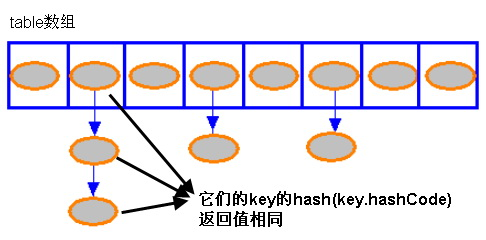# 深入讲解HashMap

2019独角兽企业重金招聘Python工程师标准>>>HashMap有很多个构造函数，不过我们比较常用的是不带参数的默认构造函数，其源代码如下：

public HashMap() {

table = new Entry[DEFAULT_INITIAL_CAPACITY];

init();

}

Entry数组每一个索引处都可以存放一个Entry链（通过下面Entry的构造函数可以发现Entry对象可以指明它的下一个对象，所以多个Entry对象可以以Entry链的形式存在）

Entry类的构造函数如下：

 Entry(int h, K k, V v, Entry<K,V> n) {

value = v;

next = n;

key = k;

hash = h;

}

next指明了下一个Entry对象
hash是该Entry对象的哈希值（Hash码）

HashMap在存入键值对时首先会根据key的hashCode() 返回值来计算 Hash 码，然后通过该Hash码计算出这个这个键值对要放在数组的哪个索引处，

 public V put(K key, V value)

{

// 如果 key 为 null，调用 putForNullKey 方法进行处理

if (key == null)

return putForNullKey(value);

// 根据 key 的 keyCode 计算 Hash 值

int hash = hash(key.hashCode());

// 搜索指定 hash 值在对应 table 中的索引

int i = indexFor(hash, table.length);

// 如果 i 索引处的 Entry 不为 null，通过循环不断遍历 e 元素的下一个元素

for (Entry<K,V> e = table[i]; e != null; e = e.next)

{

Object k;

// 找到指定 key 与需要放入的 key 相等（hash 值相同

// 通过 equals 比较放回 true）

if (e.hash == hash && ((k = e.key) == key

|| key.equals(k)))

{

V oldValue = e.value;

e.value = value;

e.recordAccess(this);

return oldValue;

}

}

// 如果 i 索引处的 Entry 为 null，表明此处还没有 Entry

modCount++;

// 将 key、value 添加到 i 索引处

return null;

}

static int hash(int h)

{

h ^= (h >>> 20) ^ (h >>> 12);

return h ^ (h >>> 7) ^ (h >>> 4);

}

indexFor(int h, int length) 方法的代码如下：

static int indexFor(int h, int length)

{

return h & (length-1);

}

void addEntry(int hash, K key, V value, int bucketIndex)

{

// 获取指定 bucketIndex 索引处的 Entry

Entry<K,V> e = table[bucketIndex];   // ①

// 将新创建的 Entry 放入 bucketIndex 索引处，并让新的 Entry 指向原来的 Entry

table[bucketIndex] = new Entry<K,V>(hash, key, value, e);

// 如果 Map 中的 key-value 对的数量超过了极限

if (size++ >= threshold)

// 把 table 对象的长度扩充到 2 倍。

resize(2 * table.length);   // ②

}

size：该变量保存了该 HashMap 中所包含的 key-value 对的数量

threshold是阀值的意思，阀值等于Entry数组长度乘以负载因子，从上面的例子可以看出当size++ >= threshold 时，HashMap 会自动调用 resize 方法扩充 HashMap 的容量。每扩充一次，HashMap 的容量就增大一倍。

HashMap 类的 get(K key) 方法代码：

 public V get(Object key)

{

// 如果 key 是 null，调用 getForNullKey 取出对应的 value

if (key == null)

return getForNullKey();

// 根据该 key 的 hashCode 值计算它的 hash 码

int hash = hash(key.hashCode());

// 直接取出 table 数组中指定索引处的值，

for (Entry<K,V> e = table[indexFor(hash, table.length)];

e != null;

// 搜索该 Entry 链的下一个 Entr

e = e.next)    // ①

{

Object k;

// 如果该 Entry 的 key 与被搜索 key 相同

if (e.hash == hash && ((k = e.key) == key

|| key.equals(k)))

return e.value;

}

return null;

}